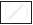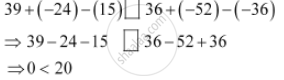Share

# Use the Sign of >, < Or = in the Box to Make the Statements True. 39 + (– 24) – (15) And 36 + (– 52) – (– 36) - CBSE Class 7 - Mathematics

ConceptIntroduction of Integer

#### Question

Use the sign of >, < or = in the box to make the statements true.

39 + (– 24) – (15)36 + (– 52) – (– 36)

#### SolutionIs there an error in this question or solution?

#### APPEARS IN

NCERT Solution for Mathematics for Class 7 (2018 to Current)
Chapter 1: Integers
Ex. 1.10 | Q: 9.4 | Page no. 5
Solution Use the Sign of >, < Or = in the Box to Make the Statements True. 39 + (– 24) – (15) And 36 + (– 52) – (– 36) Concept: Introduction of Integer.
S